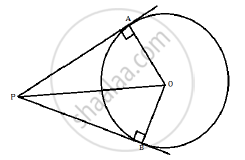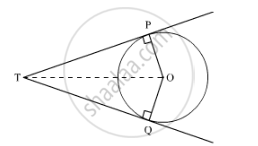# Prove that the Lengths of the Tangents Drawn from an External Point to a Circle Are Equal. - Mathematics

Prove that the lengths of the tangents drawn from an external point to a circle are equal.

Prove that the lengths of two tangents drawn from an external point to a circle are equal.

#### Solution 1

Consider the following diagram.Let P be an external point and PA and PB are tangents to the circle. We need to prove that PA = PB Now consider the triangles ΔOAP and ΔOBP

m∠A = m∠B = 90°

OP = OP [common]

OA = OB = radii of the circle

Thus, by Right Angle-Hypotenuse-Side criterion of congruence we have,

ΔOAP ≅ ΔOBP

The corresponding parts of the congruent triangles are congruent.

Thus, PA = PB

#### Solution 2Given: TP and TQ are two tangent drawn from an external point T to the circle C (O, r).

To prove: TP = TQ

Construction: Join OT.

Proof: We know that a tangent to the circle is perpendicular to the radius through the point of contact.

∴ ∠OPT = ∠OQT = 90°

In ΔOPT and ΔOQT,

OT = OT (Common)

OP = OQ (Radius of the circle)

∠OPT = ∠OQT (90°)

∴ ΔOPT ≅ ΔOQT (RHS congruence criterion)

⇒ TP = TQ (CPCT)

Hence, the lengths of the tangents drawn from an external point to a circle are equal.

Concept: Number of Tangents from a Point on a Circle
Is there an error in this question or solution?
2014-2015 (March) All India Set 3

Share